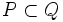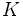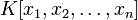# Catenary ring

This article defines a property of commutative unital rings; a property that can be evaluated for a commutative unital ring
View all properties of commutative unital rings
VIEW RELATED: Commutative unital ring property implications | Commutative unital ring property non-implications |Commutative unital ring metaproperty satisfactions | Commutative unital ring metaproperty dissatisfactions | Commutative unital ring property satisfactions | Commutative unital ring property dissatisfactions

## Definition

A commutative unital ring is termed a catenary ring or chain ring or is said to satisfy the Saturated Chain Condition if it is Noetherian and satisfies the following equivalent conditions:

• If$P < P_1 < P_2 < Q$ is a strictly ascending chain of prime ideals, and$P'$ is a prime ideal between$P$ and$Q$, then there is either a prime ideal between$P$ and$P'$ or a prime ideal between$P'$ and$Q$
• Given two prime ideals$P$ and$Q$ such that$P \subset Q$, the length of any saturated chain of primes between$P$ and$Q$ (i.e. a chain of primes in which no more primes can be inserted in between) is determined independent of the choice of chain

Note that being catenary does not guarantee that any two fully saturated chains of primes (i.e. any two chains of primes which are saturated and cannot be extended in either direction) have the same length. The problem is that the starting and ending points of the chains may differ: there may be many different maximal ideals and many different minimal prime ideals.

## Relation with other properties

### Stronger properties

Property Meaning Proof of implication Proof of strictness (reverse implication failure) Intermediate notions
polynomial ring over a field of the form$K[x]$ where$K$ is a field click here
multivariate polynomial ring over a field of the form$K[x_1,x_2,\dots,x_n]$ where$K$ is a field click here
principal ideal domain integral domain in which every ideal is a [principal ideal]] click here
universally catenary ring every finitely generated algebra over it is a catenary ring. click here
Cohen-Macaulay ring for every ideal, the depth equals the codimension click here

### Weaker properties

Property Meaning Proof of implication Proof of strictness (reverse implication failure) Intermediate notions